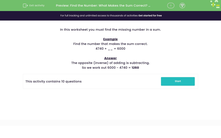# Use the Inverse Operation to Solve Addition Calculations

In this worksheet, students will find the missing number in a calculation using subtraction.Key stage:  KS 2

Curriculum topic:   Number: Addition and Subtraction

Curriculum subtopic:   Use Correct Methods to Problem Solve

Difficulty level:#### Worksheet Overview

In this activity, we will be finding the missing number in a calculation.

Example

Find the number that makes the calculation correct.

4,740 + __ = 6,000

The opposite (inverse) of adding is subtracting.

So we work out 6,000 - 4,740 = 1,260Here is another example

2,365  + ___ = 4,000

Use the inverse operation to solve.

4,000  -  2,365 = 1,635

If you remember that the inverse simply means the opposite, you'll soon be flying through the questions!

The inverse of addition is subtraction, and yes, you've guessed it, the inverse of subtraction is addition!

Let's get started!### What is EdPlace?

We're your National Curriculum aligned online education content provider helping each child succeed in English, maths and science from year 1 to GCSE. With an EdPlace account you’ll be able to track and measure progress, helping each child achieve their best. We build confidence and attainment by personalising each child’s learning at a level that suits them.

Get started